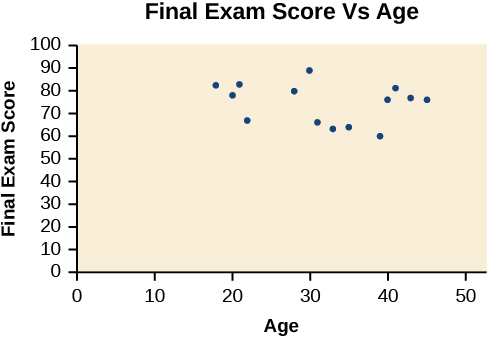# 4.3 Fitting linear models to data

 Page 1 / 14
In this section you will:
• Draw and interpret scatter diagrams.
• Use a graphing utility to find the line of best fit.
• Distinguish between linear and nonlinear relations.
• Fit a regression line to a set of data and use the linear model to make predictions.

A professor is attempting to identify trends among final exam scores. His class has a mixture of students, so he wonders if there is any relationship between age and final exam scores. One way for him to analyze the scores is by creating a diagram that relates the age of each student to the exam score received. In this section, we will examine one such diagram known as a scatter plot.

## Drawing and interpreting scatter plots

A scatter plot is a graph of plotted points that may show a relationship between two sets of data. If the relationship is from a linear model , or a model that is nearly linear, the professor can draw conclusions using his knowledge of linear functions. [link] shows a sample scatter plot.A scatter plot of age and final exam score variables

Notice this scatter plot does not indicate a linear relationship . The points do not appear to follow a trend. In other words, there does not appear to be a relationship between the age of the student and the score on the final exam.

## Using a scatter plot to investigate cricket chirps

[link] shows the number of cricket chirps in 15 seconds, for several different air temperatures, in degrees Fahrenheit Selected data from http://classic.globe.gov/fsl/scientistsblog/2007/10/. Retrieved Aug 3, 2010 . Plot this data, and determine whether the data appears to be linearly related.

 Chirps 44 35 20.4 33 31 35 18.5 37 26 Temperature 80.5 70.5 57 66 68 72 52 73.5 53

Plotting this data, as depicted in [link] suggests that there may be a trend. We can see from the trend in the data that the number of chirps increases as the temperature increases. The trend appears to be roughly linear, though certainly not perfectly so.

## Finding the line of best fit

Once we recognize a need for a linear function to model that data, the natural follow-up question is “what is that linear function?” One way to approximate our linear function is to sketch the line that seems to best fit the data. Then we can extend the line until we can verify the y -intercept. We can approximate the slope of the line by extending it until we can estimate the $\text{\hspace{0.17em}}\frac{\text{rise}}{\text{run}}.$

## Finding a line of best fit

Find a linear function that fits the data in [link] by “eyeballing” a line that seems to fit.

On a graph, we could try sketching a line. Using the starting and ending points of our hand drawn line, points (0, 30) and (50, 90), this graph has a slope of

$\begin{array}{l}\hfill \\ \begin{array}{l}m=\frac{60}{50}\hfill \\ \text{\hspace{0.17em}}\text{\hspace{0.17em}}\text{\hspace{0.17em}}\text{\hspace{0.17em}}=1.2\hfill \end{array}\hfill \end{array}$

and a y -intercept at 30. This gives an equation of

$T\left(c\right)=1.2c+30$

where $\text{\hspace{0.17em}}c\text{\hspace{0.17em}}$ is the number of chirps in 15 seconds, and $\text{\hspace{0.17em}}T\left(c\right)\text{\hspace{0.17em}}$ is the temperature in degrees Fahrenheit. The resulting equation is represented in [link] .

## Recognizing interpolation or extrapolation

While the data for most examples does not fall perfectly on the line, the equation is our best guess as to how the relationship will behave outside of the values for which we have data. We use a process known as interpolation when we predict a value inside the domain and range of the data. The process of extrapolation is used when we predict a value outside the domain and range of the data.

#### Questions & Answers

write down the polynomial function with root 1/3,2,-3 with solution
if A and B are subspaces of V prove that (A+B)/B=A/(A-B)
write down the value of each of the following in surd form a)cos(-65°) b)sin(-180°)c)tan(225°)d)tan(135°)
Prove that (sinA/1-cosA - 1-cosA/sinA) (cosA/1-sinA - 1-sinA/cosA) = 4
what is the answer to dividing negative index
In a triangle ABC prove that. (b+c)cosA+(c+a)cosB+(a+b)cisC=a+b+c.
give me the waec 2019 questions
the polar co-ordinate of the point (-1, -1)
prove the identites sin x ( 1+ tan x )+ cos x ( 1+ cot x )= sec x + cosec x
tanh`(x-iy) =A+iB, find A and B
B=Ai-itan(hx-hiy)
Rukmini
what is the addition of 101011 with 101010
If those numbers are binary, it's 1010101. If they are base 10, it's 202021.
Jack
extra power 4 minus 5 x cube + 7 x square minus 5 x + 1 equal to zero
the gradient function of a curve is 2x+4 and the curve passes through point (1,4) find the equation of the curve
1+cos²A/cos²A=2cosec²A-1
test for convergence the series 1+x/2+2!/9x3ByByBy David MartinByByByBy Lakeima Roberts